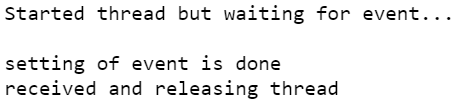Open in App
Not now

# Implement Inter Thread Communication with Event( ) Method in Python

• Last Updated : 26 Nov, 2022

Here we will start from the basics of what inter-thread communication is? Inter Thread Communication is the process of communicating requirements between one to another thread. In simple words sometimes one thread may be required to communicate to another thread depending on the requirements. This is considered as Inter Thread Communication

Event() Method: Here we talk about the Event() method, the Event object is considered or recommended as the simplest communication process or system between any threads. This system works on two conditions where the Event object is Enabled means set() or disabled means clear().

Syntax:

In the Internally Event manages the process which will be worked internally and it can be Set (enabled) or Clear (disabled) using the methods on event objects. If the threads are to be Set then all the threads are going to be executed but if the threads are to be Clear then generally all the threads become to wait for execution.

Example :

We take an example to explain how the event method is used in the implementation of inter-thread communication:

## Python3

 `#import modules` `import` `threading` `import` `time`   `if` `__name__ ``=``=` `'__main__'``:`   `    ``# initializing the event object` `    ``event_object ``=` `threading.Event()`   `# defining task` `def` `task():` `  `  `    ``print``(``"\nStarted thread but waiting for event..."``)` `    ``event_set ``=` `event_object.wait(``4``)` `    `  `    ``if` `event_set:` `        ``print``(``"\nreceived and releasing thread"``)` `    ``else``:` `        ``print``(``"\ntime is gone..."``)`     `# assigning task` `thread1 ``=` `threading.Thread(target``=``task)`   `# starting thread` `thread1.start()`   `time.sleep(``3``)` `event_object.``set``()` `print``(``"\nsetting of event is done"``)`

Output:In the above following program in which we create the event object, and then we create a thread and start it, now the thread is set the event object with the set() method and in function task() where the thread is in waiting for the state if the event is set to the thread will execute here next instruction if it was not set then the program is terminated still there is having an instruction to be executed.

Here are some general methods are used in the Event class:-

•  clear( ) Method: This method is fully opposite of the set() method, but this method also acts as a condition changer if the condition becomes False then which thread is not running or already in waiting, so they are still is in waiting for state and don’t continue their execution.
• set( ) Method: In the set() method we used it as a condition changer between threads where if the condition will True then there are much thread which was in waiting for the state they become continue their execution.
• isSet( ) Method: This isSet() method has meaning as their name suggests is set, this method simplifies that the following event that we have created are set or not set.
• wait( time ) Method: To describe the wait() method in simple words we can say that thread waits until the execution of the set() method is not done. We can use time in it if we set a certain time then the execution will stop until time overs after that it will execute still the set() of an event is remaining.

Here we will take a simple example to explain how the above methods are used throughout the entire program:

## Python3

 `# import time module` `import` `time`   `# import threading module` `import` `threading`   `class` `product:` `  `  `  ``def` `buyer(``self``):` `    ``print``(``'John consumer is wait for product'``)   ` `    ``print``(``'...............'``)` `    ``event_object.wait()    ` `    ``print``(``'got product'``)       ` `  `  `  ``def` `seller(``self``):   ` `    ``time.sleep(``5``)` `    ``print``(``'Tom producer producing items'``)  ` `    ``print``(``'tom goes to retailer'``)` `    ``event_object.wait()` `    `  `  ``def` `retailer(``self``):` `    ``time.sleep(``10``)` `    ``print``(``'retailer found that product and directly send to buyer'``)` `    ``event_object.``set``()` `          `    `# class object      ` `class_obj ``=` `product()`   `# setting event object` `if` `__name__``=``=``'__main__'``:` `  ``event_object ``=` `threading.Event()`   `# creating  threads` `T1 ``=` `threading.Thread(target``=``class_obj.buyer)` `T2 ``=` `threading.Thread(target``=``class_obj.seller)` `T3 ``=` `threading.Thread(target``=``class_obj.retailer)`   `# starting threads` `T1.start()` `T2.start()` `T3.start()`

Output: